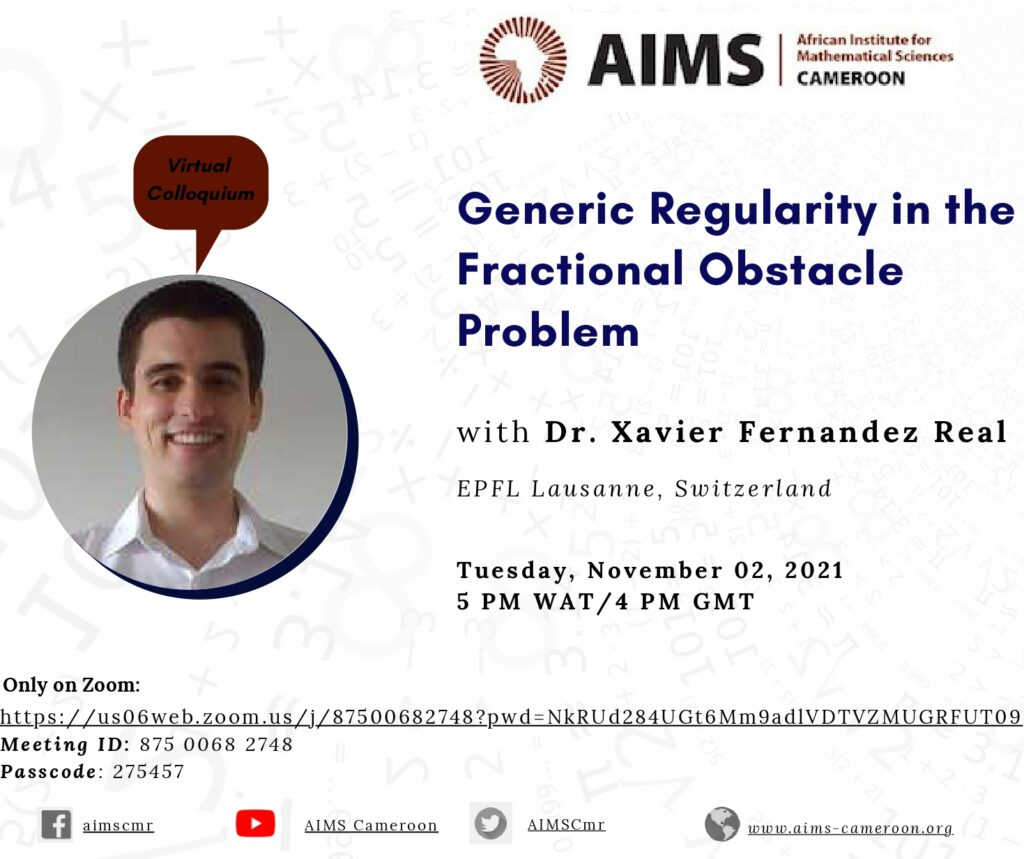November 2, 2021

# Generic Regularity in the Fractional Obstacle Problem

The fractional obstacle problem in $\mathbb R^n$ with obstacle $\varphi\in C^\infty(\mathbb{R}^n)$ can be written as

$\min\{(-\Delta)^s u , u-\varphi\} = 0,\quad\textrm{in }\quad\mathbb{R}^n.$ The set $\{u = \varphi\} \subset \mathbb{R}^n$ is called the contact set, and its boundary is the free boundary, an unknown of the problem.

The free boundary for the fractional obstacle problem can be divided between two subsets: regular points (around which the free boundary is smooth, and is $n-1$ dimensional) and degenerate points. The set of degenerate points, even for smooth obstacles, can be very large (for example, with infinite $\mathcal{H}^{n-1}$ measure). In joint work with X. Ros-Oton, we show, however, that generically solutions to the fractional obstacle problem have a lower-dimensional degenerate set. That is, for almost every solution (in an appropriate sense), the set of degenerate points is lower-dimensional.